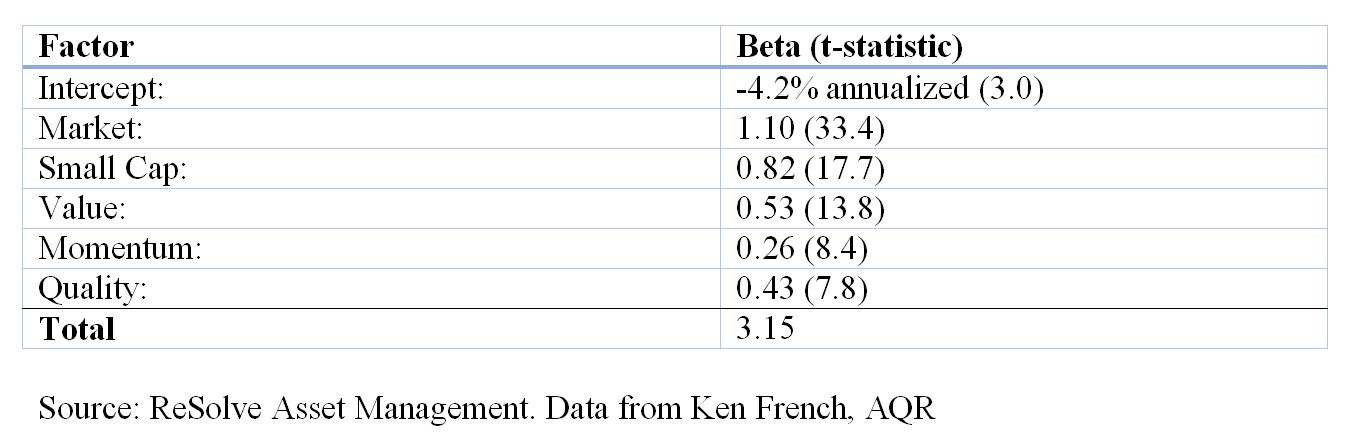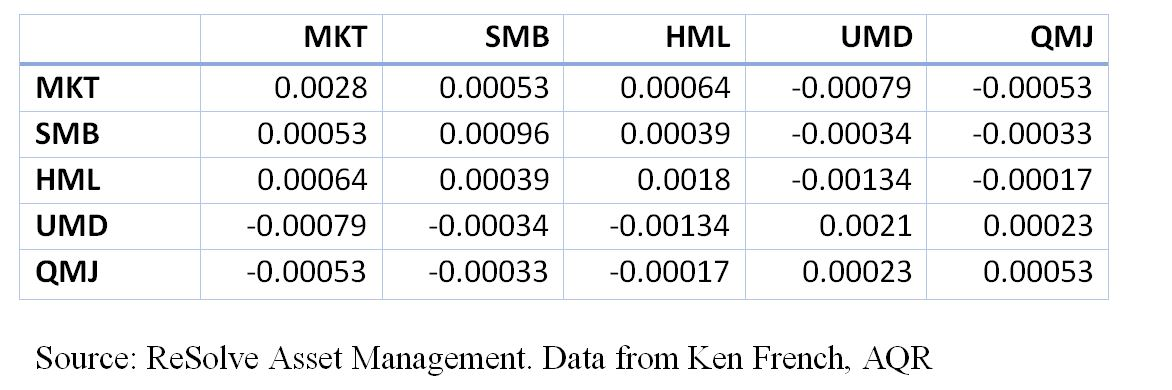# Multi-Factor Indexes: More Bang for Your Buck

Many advisors are unsure whether introducing factor-tilt ‘smart beta’ strategies into portfolios will improve client outcomes. In fact, some factor tilt portfolios appear to provide the equivalent of levered exposures to a diverse set of alternative risk premia. This is because factor tilt portfolios may contain much greater than 100% exposure to several risk factors in aggregate.

Consider the iShares S&P 600 Small-Cap Value Index ETF which is constructed to hold small-cap companies with high book-to-market, earnings-to-price, and sales-to-price ratios. Via simple back-tested regression on the underlying index back to inception in 1997, we find that it offers the following factor exposures, which are all significant at the 0.1% level:It is useful to think about the factor betas in Table 1 as weights in a synthetic portfolio. Given historical data from Ken French (MKT, SMB, HML and UMD) and AQR (QMJ), and using monthly return data, we calculate the following premia and weighted excess return:The expected total excess arithmetic return from all factor exposures would be the weighted sum of the factor exposures and the premia, plus the intercept:

1.1*7.6%+0.82*2.7%+.53*4.5%+.26*8.3%+.43*4.2%-4.2% = 12.7%.

Given that the index implies over 300% exposure to risk factors, one might expect the portfolio to exhibit much higher volatility than a market capitalization weighted index. If the factors were all highly correlated, the portfolio would have a volatility approximately equal to the weighted average volatility of all exposures:

1.1*18%+.82*11%+.53*14%+.26*16%+.43*8% = 43.8%.

This would have meaningful implications in terms of compound return, as the implied compound annual return of the portfolio would be the arithmetic mean of 12.7% minus a volatility decay factor equal to half the portfolio variance: 0.127 – 0.5 * 0.438^2 = 3.1%. Compare this to the implied compound return of the market factor alone: 7.6% – 0.5 * 0.18^2 = 6%.

However, the factors are not highly correlated. In fact, the average off-diagonal pairwise correlations of the five factors in Table 1. is just -0.14. As such, we would expect the volatility of the portfolio to be less than the weighted average volatility of the factors. In fact, we can estimate expected volatility of the portfolio (sigma_p) from the following formula:

sigma_p = sqrt(w^T Sigma w),

where w= the factor exposures from Table 1. and Sigma is the covariance matrix of the factors:Thus, the implied expected portfolio volatility would be about 25%. The compound excess return would be 12.7% – 0.5*.25^2 = 9.6, which now compares quite favorably to the capitalization weighted market factor. Moreover, the Sharpe ratio of the factor-tilted Index is 12.7%/25%=0.51, compared with the market’s Sharpe ratio of .42.